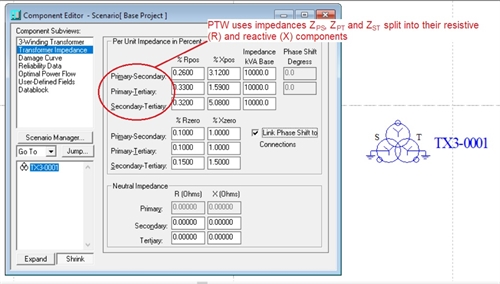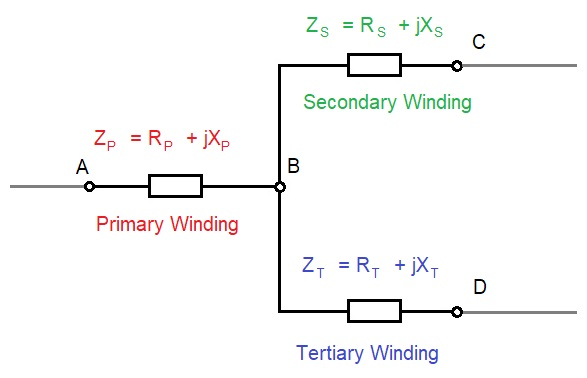CEE Relays Ltd

Tel:   +44 1753 576477    Fax: +44 1753 825661
FAQs: How do I enter impedance data for a 3-winding transformer?
Three-winding transformers are sometimes specified on datasheets with separate impedances for the primary (P) winding, the secondary (S) winding and the tertiary (T) winding.  These may appear on your datasheet as ZP, ZS and ZT respectively.

However, power systems studies require this information to be expressed in a different way.  When entering transformer impedance data, the required values are ZPS, ZPT and ZST.  Why is this and how do I convert the information from one form to another?(Left): Screenshot from PTW.
In the background is a three-winding transformer that has been created in the "One-Line" screen; in the foreground is the "Component Editor" screen where the transformer impedance data is entered.
Transformer equivalent circuit:
The 3-winding transformer equivalent circuit consists of three impedances connected in star formation.  These represent the impedances of the primary, secondary and tertiary windings.
(Right): Equivalent impedance circuit.
From this we can see that, for example, the primary impedance ZP is the impedance between points A and B.
The primary-secondary impedance (ZPS) is the impedance between points A and CWe can therefore derive six formulae to calculate the transformer equivalent impedances:
1. Primary-secondary impedance, ZPS = ZP + ZS
2. Primary-tertiary impedance, ZPT = ZP + ZT
3. Secondary-tertiary impedance, ZST = ZS + ZT
4. Primary impedance, ZP = ( ZPS + ZPT - ZST ) / 2
5. Secondary impedance, ZS = ZPS - ZP
6. Tertiary impedance, ZT = ZPT - ZP
The first three formulae may be used to calculate the impedances for entering into PTW (if only primary, secondary and tertiary impedance data has been provided).  Formulae 4, 5, and 6 are provided for information only.